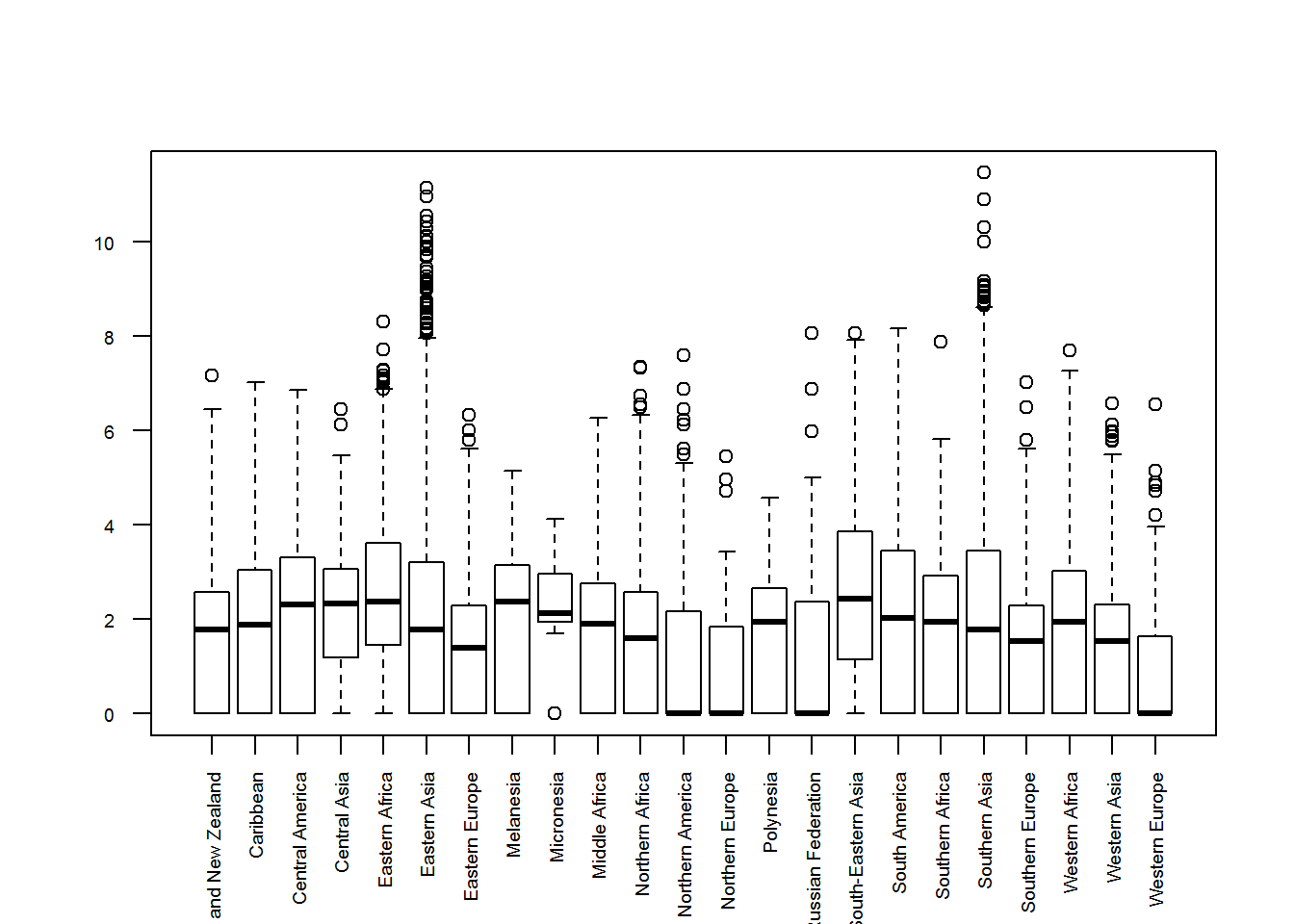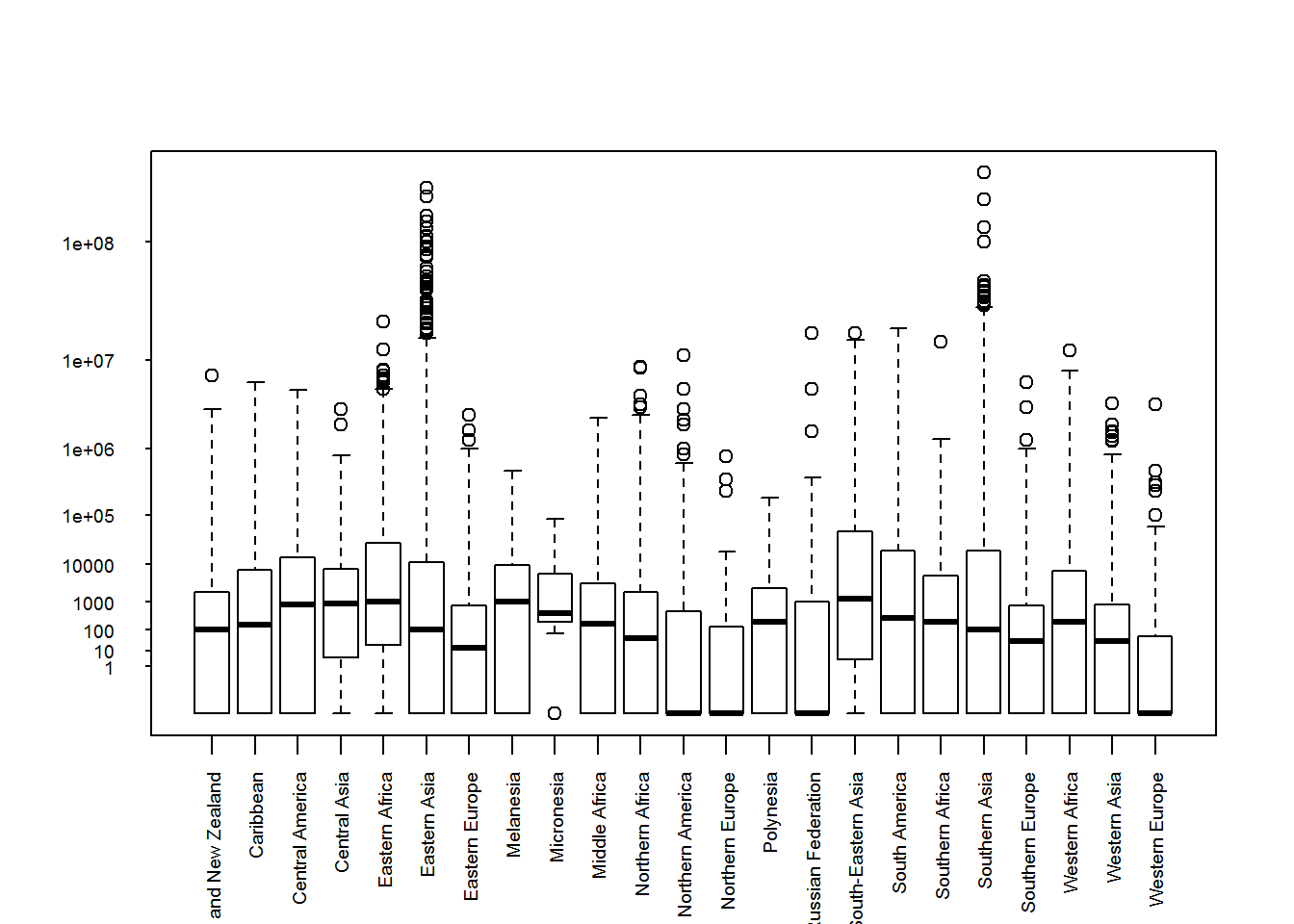BIS-Fogo

Site Tools

en:learning:schools:s01:code-examples:ba-ce-09-02

C09-2 - Generic axis labeling

The following plotting examples will revisit R’s generic plotting functions and pimp them up a little bit.

The underlaying example data is taken from our combined natural disaster and Ebola data set which is loaded in the background into the data frame called df. We will generally not interpret the plots but just look at formal aspects.

In a previous example, we have made a box plot with square root transformed data. The boxplot looked like this:

df$total_affected_sqrt3 <- df$total_affected^(0.5)^3
boxplot(total_affected_sqrt3 ~ region, data = df,
las=2, cex.axis=0.6)The problem with that plot is that the labels at the y axis are no longer equal to the actual values but to the third power of the square root. Since this is hard to convert back without a calculator, let’s produce a plot which has the same scaling as this one but modified tics (i.e. the points where a label is drawn) and lable values.

The tutorial has been created using R’s Markdown language and hence we can not add something to the plot in different code sections. Therefore we will show that it looks like now and explain it below the plot.

# plot the boxplot without y axis tics and lables
boxplot(total_affected_sqrt3 ~ region, data = df,
las=2, cex.axis=0.6,
yaxt="n")

# get maximum value as power of 10 (by counting digits of the max value)
# and create a vector of these powers starting with 10^0
ndigits <- nchar(as.character(max(df\$total_affected)))
ylabls <- 10^(0:ndigits)
print(ylabls)
##   1e+00 1e+01 1e+02 1e+03 1e+04 1e+05 1e+06 1e+07 1e+08 1e+09
# transform the power of then values above analogous to the data values
ytics <- ylabls^(0.5)^3

# add axis tics and labels to the plot
axis(2, at=ytics, labels=ylabls, las=2, tck=-.01, cex.axis=0.6)If you look at the code above you will notice that the first thing that has changed is the yaxt argument in the boxplot function. This argument controls if yaxt lables and tics are drawn and by setting it to “n”, no axis tics etc. will be put into the plot in the first place.

The second block of the code counts the digits of the maximum value of our data set. Therefore it is necessary to convert the number to a character. The number of digits gives us the highes power of 10 which is necessary to describe the data.

After we now what power of ten just above the maximum value of our data, we create a vector with powres of ten starting from 1 (i.e. power = 0),

Now we have our lables (i.e. values of the power of 10). To place them at the correct position in the plot, we have to define the position of the tics in the square root transformed plot. Therefore we apply the same square root transformation to the tic values as we have applied to the data set.

Finally, we draw the axis tics and lables using the axis function.